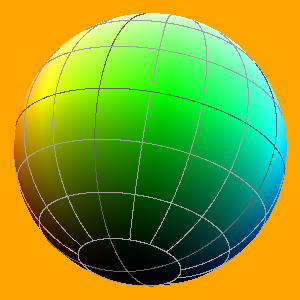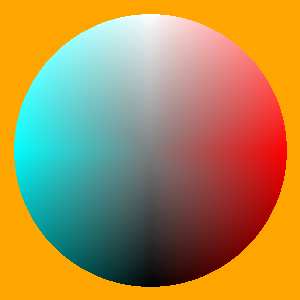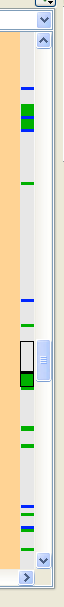# The Colourball

For my artwork I need of course colours. And there exists the colour-circle of Goethe. But it has not all colours. Then there are the RGB colours. RGB stands for red, green, blue. But by means of RGB you cannot address the colours intuitively and continuously varied, because the three values goes from 0 to 255 and then they stop.

Therefore I invented the colourball.

The idea is very simple. The colourball has the radius of 1. On its surface are the pure colours. The north pole is white, the south pole is black, the equator has got the colours of the rainbow like the colour-circle. Complementary colours lay opposite, colours on the same latitude circle have always the same brightness, even if they lay within the ball, the pole-axle has no colour and has got the grey-scale.

And so I can aim with the polar coordinates each colour exactly and vary without jumps like at the RGB colours.

The Colourballfront view with north pole (left) and back view with south pole (right)

Colourball - insidecut along the zero meridian

I wrote in python a transformation with it I can convert the 3 polar coordinates of the colourball in RGB and back again. And because this has more to do with mathematics, I put this under the category "Mathematik".

Here is the python-script:
Hpr stands for:   Hue, polar angle, radius
H   goes from 0 to 360 and means the value of the colour
p   goes from -1 (black) to 1 (white); 0 means the middle brightness
r   goes from 0 to 1; 0 is the middle-grey, 1 are the pure colours

RGB stands for red, green, blue, the values go from 0 to 255

If you want to have the polar angle in degrees from -90 to 90, just enter the row phi = sin(radians(phi)) or phi = phi/90 (as you like)

def RGBHpr(R, G, B):
r, g, b = R/255, G/255, B/255
maxc = max(r, g, b)
minc = min(r, g, b)
ra = 2*max(abs(0.5-r), abs(0.5-g), abs(0.5-b))
phi = (maxc+minc-1)/ra
if minc == maxc:
h = 0
else:
rc = (maxc-r) / (maxc-minc)
gc = (maxc-g) / (maxc-minc)
bc = (maxc-b) / (maxc-minc)
if r == maxc:
h = bc-gc
elif g == maxc:
h = 2.0+rc-bc
else:
h = 4.0+gc-rc
h = (h/6.0) % 1.0
H = 360*h
return H, phi, ra

def HprRGB(H, phi = 0, ra = 1):
if phi == -1 and ra == 1:
return 0, 0, 0
if phi > 0:
v = (1+ra)/2
s = ra*(1-phi)/v
else:
v = (ra+2*phi*ra+1)/2
s = ra*(1+phi)/v
h = H%360/360
if s == 0.0:
R, G, B = 255*v, 255*v, 255*v
else:
i = int(h*6.0)
f = (h*6.0) - i
p = v*(1.0 - s)
q = v*(1.0 - s*f)
t = v*(1.0 - s*(1.0-f))
i = i%6
v = 255*v
t = 255*t
p = 255*p
q = 255*q
if i == 0:
R, G, B = v, t, p
if i == 1:
R, G, B = q, v, p
if i == 2:
R, G, B = p, v, t
if i == 3:
R, G, B = p, q, v
if i == 4:
R, G, B = t, p, v
if i == 5:
R, G, B = v, p, q
return int(R), int(G), int(B)

Sometimes it is better to take a Colouronion instead of the Colourball. Then the radius does not go radial from the middle-grey but perpendicular from the pole-axle in percent of the pure colour, so that the brightness stays the same if you vary the radius.

Here is the Colouronion-script:

def fz(H, q = 0, n = 1):
if q == 0 and n == 0:
return 127,127,127
r = (q**2*(1 - n**2) + n**2)**0.5
p = q/r
return HprRGB(H, p, r)

def RGBfz(R, G, B):
H, phi, ra = RGBHpr(R, G, B)
q = ra*phi
n = ra*((1-phi**2)/(1-ra**2*phi**2))**0.5
return H, q, n

q   goes from -1 to 1 and stands for the polar-angle;
n   goes from 0 to 1.

## The eric scrollbar

And because we are at the theme python:

I had the idea for the searchhit-indicator in the scrollbar of the IDE eric. Detlev Offenbach was so kind and talented to implement the idea.

The Scrollbarwith searchhit-indicator

If you put the cursor within the integrated development environment on a digit, name or variable, than within the scrollbar all places are marked in blue, where the digit, name or variable occurs in the script. So you can see immediately, where it is and how often it occurs. And with an easy drag with the mouse you get to the place. This is fast and secure.

In the past you often overlooked variables if you had to change something while programming. This caused very often mistakes in the programme.

It is only a question of time, until the other IDEs will copy it. It is very useful.